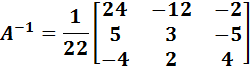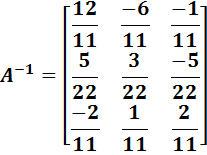# Chapter 2 : Matrix

### Topics covered in this snack-sized chapter:

#### Matrix arrow_upward

• A matrix is a rectangular array of numbers, symbols, or expressions, arranged in rows and columns. The individual items in a matrix are called its elements or entries.
• A set of mn numbers arranged in the form of an ordered set of m rows and n columns is called m × n matrix (to be read as m by n matrix).
• A matrix with m rows and n columns can be written as:
•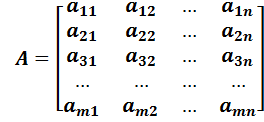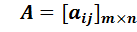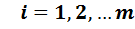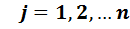• Where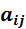represents the element at the intersection of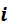th row and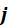th column.

• #### Types of Matrices arrow_upward

###### Square Matrix

• A matrix in which the number of rows is equal to the number of columns is called a Square Matrix.
• Thus a matrix A with m rows and n columns will be a square matrix if

m = n.

###### Diagonal Elements

• In a square matrix all those elementsfor which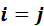that is, all those elements which occur in the same row and same column namely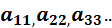are called the diagonal elements.

• ###### Diagonal Matrix

• A square matrix A is said to be a diagonal matrix if all its non-diagonal elements be zero.
•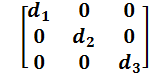###### Scalar Matrix

• A diagonal matrix whose all the diagonal elements are equal, is called a scalar matrix.
•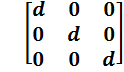###### Unit or Identity Matrix

• A square matrix A all of whose non-diagonal elements are zero and all the main diagonal elements are unity.

• ###### Example: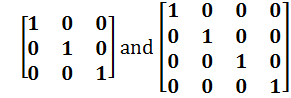In general for a unit matrix,

•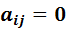For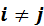and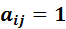for• ###### Zero Matrix or Null Matrix

• Any m × n matrix in which all the elements are zero is called a zero matrix or a null matrix of the type m × n and is denoted by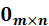•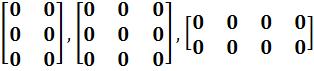###### Symmetric Matrix

• A symmetric matrix is a square matrix that satisfies AT = A where AT represents the transpose such that aij = aji .
• It also implies A-1 A = I where I is an identity matrix.
• Let A be the symmetric matrix in which
•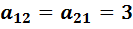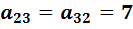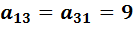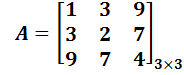###### Row Matrix

• Row Matrix is a matrix with only one row.
• For example:
•     [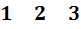]

###### Column Matrix

• A matrix which has only one column is called a Column Matrix.
• For example:
•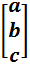###### Equal Matrix

• Two matrices are said to be equal if they have the same order and their corresponding entries are equal.
• For example:
•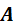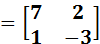and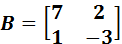are equal matrix because both the matrix A and B have same order and same corresponding entries.

• #### Properties of Matrix Addition arrow_upward

• A + B = B + A

•     A + (B + C) = (A + B) + C

#### Properties of Matrix Multiplication arrow_upward

• Multiplication of Matrices is distributive with respect to addition of matrices.
•     A (B + C) = AB + AC

• Matrix Multiplication is associative if conformability is assured.
• A(BC) = (AB)C
• The multiplication of Matrices is not always commutative.
• AB is not always equal to BA.
• Multiplication of a Matrix A by a null matrix conformable with A for multiplication is a null matrix.
• A0 = 0
• If AB = 0 then it does not necessarily mean that either A = 0 or B = 0 or both are 0.

• ###### Example: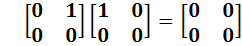###### Multiplication of Matrix A by a Unit Matrix

• Let A be a (m × n) matrix and I be a square unit matrix of order n, so that A and I are conformable for multiplication then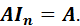• #### Transpose of a Matrix arrow_upward

• If A be a given matrix of the type m × n then the matrix obtained by changing the rows of A into columns and columns of A into rows is called Transpose of matrix A and is denoted by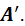• As there are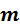rows intherefore there will becolumns in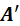and similarly as there are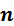columns in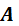therefore there will berows in• ###### Example: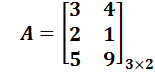• Then
•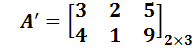#### Properties of Transpose arrow_upward

• (A')' = A
• (KA)'= KA’, K being a scalar
• (A + B)' = A' + B'
• (AB)' = B'A'
• (ABC)' = C'B'A'

• #### Solution of Linear Equations arrow_upward

• Consider the set of equations
•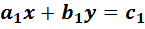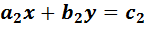Or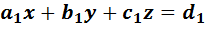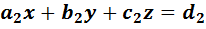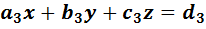• The above sets of equations can be conveniently written in matrix form as shown below:
•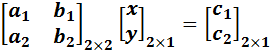• That is AX = B
• Or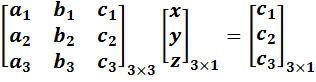• That is AX = B
• In the above, the matrixis called “coefficient matrix”.
• If the above equations have a solution, we say that they are consistent and have either a unique solution or infinite solutions.
• In case they do not have any solution, we can say that the system of equations is inconsistent.

• ###### Example: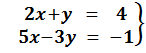have a unique solution.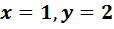can be verified by solving them.

• Here the coefficient matrix is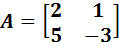and

|A| = -6 - 5 = -11 ≠ 0

• Matrix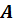is non-singular and its inverse exists.
• In this case, we will have a unique solution. The above equation can be written in matrix form as: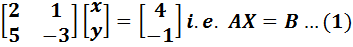• Where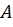is a non-singular matrix as: |A| = -11 and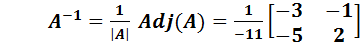.

• Multiplying both sides of (1) by A-1 , we get: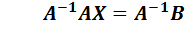• But A-1 A = I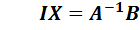• Therefore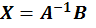or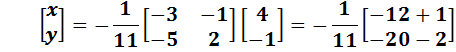Or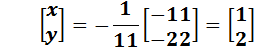Therefore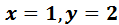#### Matrix Applications arrow_upward

###### Determinant of a Matrix

• A determinant is a real number associated with every square matrix.
• The determinant of a square matrixis denoted by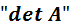or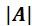.

•  Determinant of a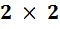matrices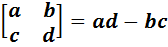#### Minors of a Matrix arrow_upward

• A minor for any element is the determinant that results when the row and column of that element are deleted.
• For the matrix shown below (Note that R1 is row 1 and C1 is column 1).

•  C1 C2 C3 R1 1 4 3 R2 0 5 2 R3 3 6 1

• Minor for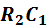(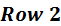,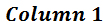, deleted) is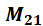:

•  C2 C3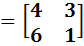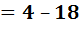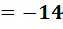R1 4 3 R3 6 1

#### Matrix of Minors arrow_upward

• The matrix of minors is the square matrix where each element is the minor for the number in that position.

•  C1 C2 C3 R1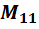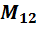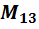R2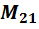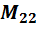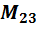R3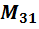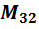#### Co-factor of a Matrix arrow_upward

• Co-factor for any element is either the minor or the opposite of the minor, based on the element's position in the original Determinant.
• If the row and column of the element add up to an even number.
• The co-factor is the same as the minor.
• If the row and column of the element add up to an odd number.
• The co-factor is the opposite of the minor.

#### Co-factor Sign Chart for a 3×3 Matrix arrow_upward

 C1 C2 C3 R1 + - + R2 - + - R3 + - +

#### Adjoint of a Matrix arrow_upward

• The matrix formed by taking the transpose of the co-factor matrix of a given matrix.

• #### The Inverse of a 3×3 Matrix arrow_upward

• It can be found in 6 steps as shown below:
• Find the matrix of minors.
• Turn it into a matrix of co-factors (by changing the sign of the odd elements).
• Find the adjoint by transposing the matrix of co-factors (Columns becomes rows and rows becomes columns).
• Find the determinant of the matrix.
• Divide the adjoint of the matrix by the determinant of the matrix.
• The final result is a matrix inverse.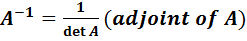or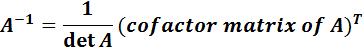###### Example:

• Consider the matrix
•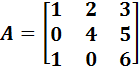• The co-factor matrix for A is: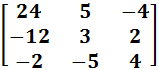•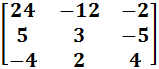•